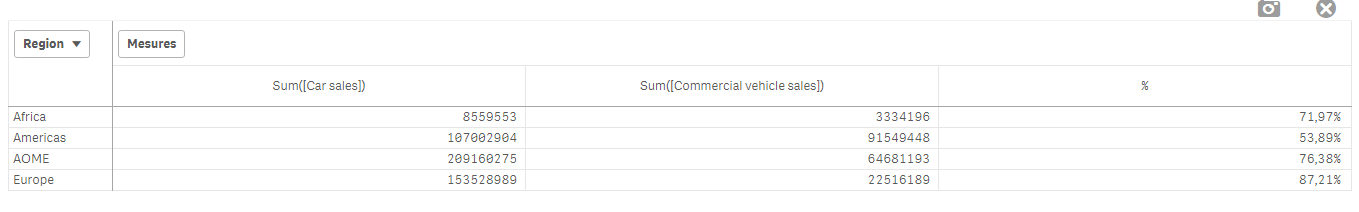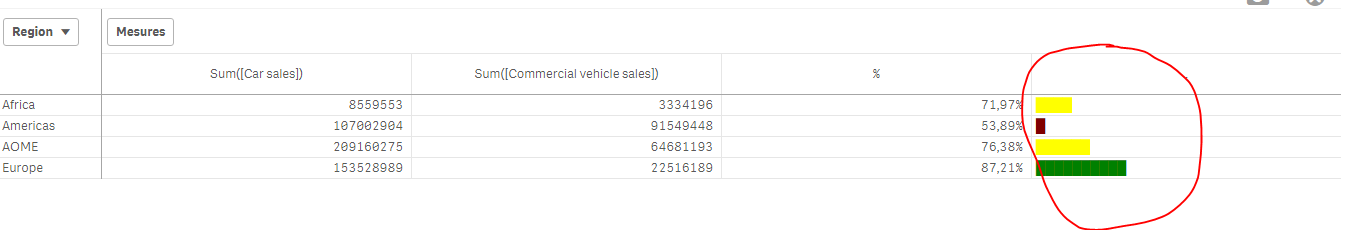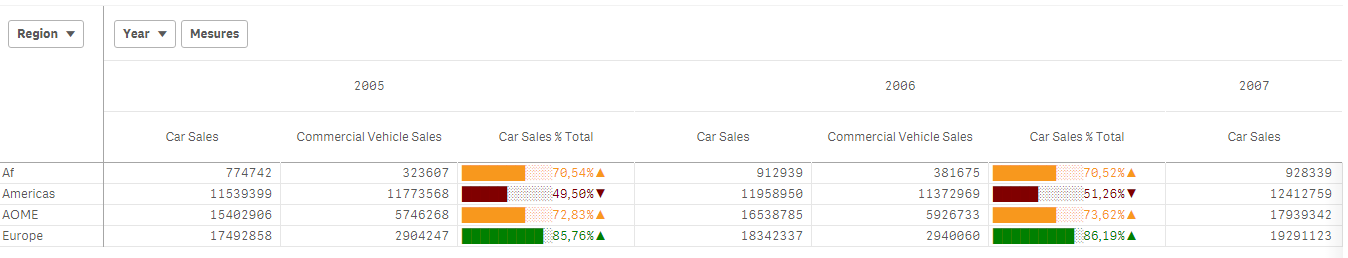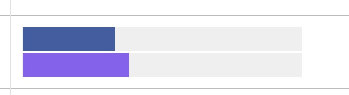# Qlik Sense Enterprise Documents & Videos

Documents & videos about Qlik Sense.

Announcements
BARC’s The BI Survey 19 makes it official. BI users love Qlik. GET REPORT

# Creating Mini Chart in Qlik Sense tablesPartner

## Creating Mini Chart in Qlik Sense tables

Hi all,

Mini Charts have always been a good feature in QlikView and It's really 'frustrating' that this has not been added (yet) in Qlik Sense.

With hopes that the Qlik Team will think about such improvements for the next releases, let's discuss here how to create our own mini chart in a Qlik Sense Table.

The following expressions might seem 'complicated' at first sight, but remember that It will be generic.

Thus, their use will be very easy.

Let's begin :

Suppose we have:

As a dimension: OICA Region

As measures:

Sum(Sales)

Sum("Commercial Vehicle Sales")

Sum(Sales) / (Sum(Sales)+Sum("Commercial Vehicle Sales"))

Here is our current table:Now, we want to add, a mini 'bar' to represent the %:

How to do so:

As an expression:

=repeat('█',

rangemax(rangemin( ceil(((column(1)/column(2))-1)*2.5),10),0)

)

With that, we repeat the square sign and precise the limits.

And as a text Color expression:

if( (sum([Car sales])/(sum([Car sales])+sum([Commercial vehicle sales])))>=0.8,Green(),

if((sum([Car sales])/(sum([Car sales])+sum([Commercial vehicle sales])))>=0.7,Yellow(),

Red()))

Result:We can also Add the % in front of the bar by adding it in the expression:

From :

=repeat('█',

rangemax(rangemin( ceil(((column(1)/column(2))-1)*2.5),10),0)

)

To:

=repeat('█',

rangemax(rangemin( ceil(((column(1)/column(2))-1)*2.5),10),0)

) & num((sum([Car sales])/(sum([Car sales])+sum([Commercial vehicle sales]))),'# ##0,00%')

Result:You can even alter it as follow:

repeat(chr(09608),round( sum([Car sales])/(sum([Car sales])+sum([Commercial vehicle sales])) * 10))

&repeat(chr(09617),10-round( sum([Car sales])/(sum([Car sales])+sum([Commercial vehicle sales])) *10))

&num((sum([Car sales])/(sum([Car sales])+sum([Commercial vehicle sales]))),'# ##0,00%')

&if(sum([Car sales])/(sum([Car sales])+sum([Commercial vehicle sales]))>0.7,'▲','▼')

result:Now, let's suppose we want to add another mini chart, a trend one, to see the % expression by Year:

If it has increased or decreased from a year to the next one.

How to do so?

Expression:

=concat(

aggr(

if((sum([Car sales])/(sum([Car sales])+sum([Commercial vehicle sales]))) > above((sum([Car sales])/(sum([Car sales])+sum([Commercial vehicle sales])))),

'▀',if((sum([Car sales])/(sum([Car sales])+sum([Commercial vehicle sales]))) < above((sum([Car sales])/(sum([Car sales])+sum([Commercial vehicle sales])))),'▄',' ')

)

,[OICA region], (Year,(NUMERIC, ASCENDING))

)

,'',[OICA region])

Wirh:

Red: Our Dimension

Green: The trending dimension

Blue: our expression compared to the previous one (by year, the trending expression)

If expression by Year > expression Previous Year then , Square Up

If expression by Year < expression Previous Year then , Square down

Result:Special thanks to blog Von Heldendaten in which I found this:

QlikView + Qlik Sense Blog von Heldendaten: Qlik Sense Calendar Measures & "Minicharts" in Tabellen.

Hope you like it !

Omar,

Tags (2)Partner

Try the simplest example I could imagine(it works on my desktop):

[data]:

RowNo() as ID

AUTOGENERATE 3;

and then in the table with dimension ID use the expression repeat('█',ID).

Contributor

Hi Maria,

I am new in Qlik Sense and I don't really understand what you mean.

Can you atash me the example?

Thank you,

Magdalena

Contributor

Hi Omar, can i change the orientation of the bars to be vertical?

New Contributor II

Hi

It works like a  charm.

The only issue is that code

repeat(chr(09608),round( sum([Car sales])/(sum([Car sales])+sum([Commercial vehicle sales])) * 10))

&repeat(chr(09617),10-round( sum([Car sales])/(sum([Car sales])+sum([Commercial vehicle sales])) *10))

&num((sum([Car sales])/(sum([Car sales])+sum([Commercial vehicle sales]))),'# ##0,00%')

&if(sum([Car sales])/(sum([Car sales])+sum([Commercial vehicle sales]))>0.7,'▲','▼')

The two bars are not overlapping but they are in sequence.

Thanks again for the code/example!Contributor

Hi Omar,

Thanks for the post, It helped me a lot.

I have one requirement on the same:

Can we have multiple color in the single row for different bars? I have combined two bars in a row using

repeat('▀',round( sum([Car sales])/(sum([Car sales])+sum([Commercial vehicle sales])) * 10))

& repeat('▀',10-round( sum([Car sales])/(sum([Car sales])+sum([Commercial vehicle sales])) *10))

now what I need is to color both bars with different colors, is this possible to do?

Regards,

Tauceef

New Contributor III

Hi All,

Is there any way to show the negative values in above mini bar chart?

Sabahat

Contributor III

Hi there,

first i wanted to say i really enjoyed your post and the creativity it provides/provoke,

I do have a question tho,

I am using the last version of sense (sept') and i'm not sure if its the version's fault but i encounter a problem with sorting when using your expression to create the whiskers chart.

I'm not sure if its the sorting of the aggr() function or not but what happens is

that the string with ASCII block is sorted so that all the '▀'  come first and then comes all the '▄' , somthing like that: '▀' .

any ideas?

I'v tried changing the sort order of the field itself in the chart properties but it didn't change anything

thanks

WizardoLuminary

Magic Thanks

Contributor

omarbensalem‌ Thank you for the post.

Any thoughts on showing 2 bars like this :Partner

Hi Daniel,

I think the concat() function of sort_weight should be the trend dimension, instead of the table dimension.

=concat(

aggr(

if((sum([Car sales])/(sum([Car sales])+sum([Commercial vehicle sales]))) > above((sum([Car sales])/(sum([Car sales])+sum([Commercial vehicle sales])))),

'▀',if((sum([Car sales])/(sum([Car sales])+sum([Commercial vehicle sales]))) < above((sum([Car sales])/(sum([Car sales])+sum([Commercial vehicle sales])))),'▄',' ')

)

,[OICA region], (Year,(NUMERIC, ASCENDING))

)

,'', Year)

Regards,

Fung

Version history
Revision #:
1 of 1
Last update:
‎2017-03-28 05:27 AM
Updated by: